Latest SSC jobs   »   Mathematics Quiz For RRB NTPC :...

# Mathematics Quiz For RRB NTPC : 25th January 2020 | Trigonometry , Algebra And Geometry

Q1.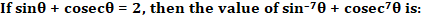Q2. A man has some hens and some cows. If the total number of heads of hens and cows together is 50 and the nunber of feet of hens and cows together is 142, then the number of cows is:
(a) 21
(b) 25
(c) 27
(d) 29

Q3. In a class, average height of all students is ‘a’ cms. Among them, average height of 10 students is ‘b’ cms and the average height of the remaining students is ‘c’ cms. Find the number of students in the class. (Here a > c and b > c)
(a) (a(b – c))/(a – c)
(b) ((b – c))/((a – c))
(c) (b – c)/(10(a – c))
(d) (10(b – c))/(a – c)

Q4.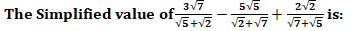(a) 0
(b) 1
(c) 5
(d) 6

Q5.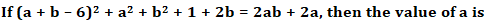(a) 7
(b) 6
(c) 3.5
(d) 2.5

Q6. Two circles of radii 17 cm and 8 cm are concentric. The length of a chord of greater circle which touches the smaller circle is:
(a) 15 cm
(b) 16 cm
(c) 30 cm
(d) 34 cm

Q7. The least six digit number which is a perfect square is
(a) 100489
(b) 100000
(c) 100256
(d) 100225

Q8. The percentage change of a number when it is first decreased by 10% and then increased by 10% is:
(a) 0.1% increase
(b) 1% decrease
(c) 0.1% decrease
(d) No changes

Q9. Find the angular elevation of the sum when the shadow of a 15m long pole is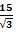metres
(a) 45°
(b) 60°
(c) 30°
(d) 90°

Q10.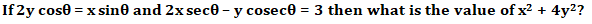(a) 4
(b) 1
(c) 2
(d) 5

#### Solutions: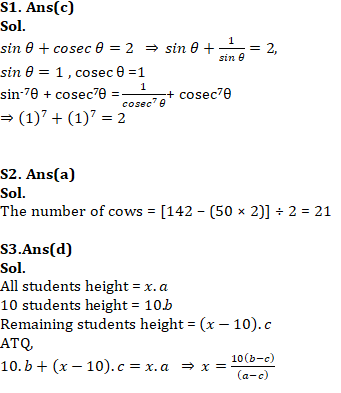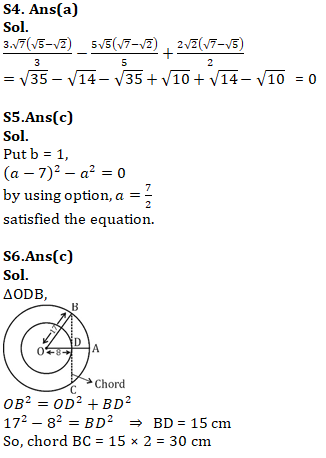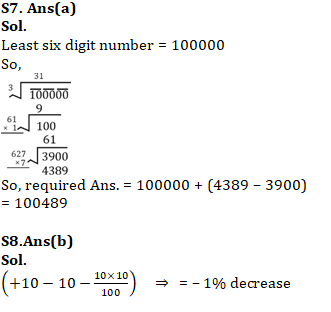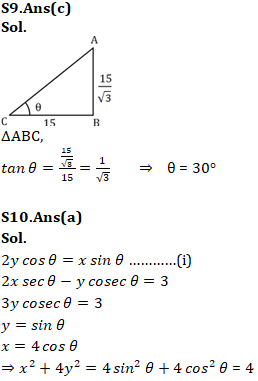Important Links for RRB NTPC Recruitment 2019
RRB NTPC Previous year Cut Off | 1st & 2nd Stage Examination
RRB NTPC Recruitment 2019: Check FAQs
RRB NTPC Exam Pattern 2019 – Check Here
RRB NTPC Previous Year Exam Analysis
RRB NTPC Exam Syllabus#### Congratulations!General Awareness & Science Capsule PDF